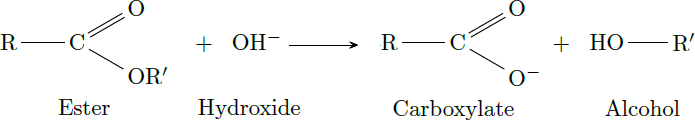# Saponification

Chemistry Level 2An ester in aqueous solution is split by the addition of sodium hydroxide in a carboxylate and an alcohol (saponification). After a period of two minutes, the ester concentration has decreased by 20%.

How long does it take to convert 80% of the ester?

Details: The saponification is a second-order reaction with the reaction rate $r = k_2 \cdot [\text{Ester}] \cdot [\text{OH}^-],$ where $k_2$ is the reaction constant. The initial concentrations of ester and hydroxide are both equal: $[\text{Ester}] _0 = [\text{OH}^-]_0.$

×